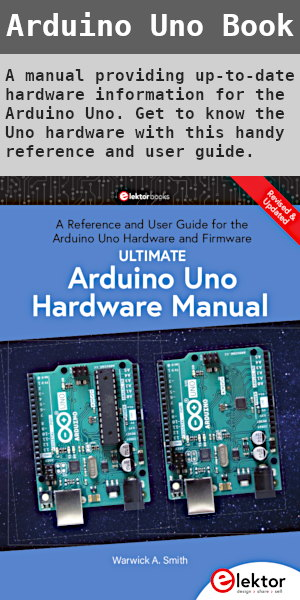# Arduino LCD Voltmeter with 4 Channels

Created on: 27 May 2013

Analog channels A2 to A5 on an Arduino Uno are used to measure four different voltages. The measured voltages are displayed on a 16 character by 2 line LCD.

The four channel Arduino multimeter can measure four independent DC voltages that can each be in the range of 0 to 50V.

Voltages are displayed with one decimal place, e.g. 5.3V, 12.8V, etc.

This video shows the Arduino voltmeter being used to measure the voltage of four batteries. Each battery has a different voltage.

Can't see the video? View on YouTube →

## How the Voltmeter Works

Each channel of the Arduino voltmeter has a pair of resistors that form a voltage divider. The voltage divider scales down the input voltage to a range that the Arduino can measure. Code running on the Arduino calculates the actual voltage and displays the result on the LCD.

How the voltage divider works, precautions and voltage range are explained in the article Measuring DC Voltage using Arduino. The Arduino voltmeter uses the same resistor values as the article.

## Arduino Voltmeter Circuit Diagram

The LCD used in the circuit has the pin numbering shown in the LCD component information from the beginner's tutorial on this website.

It is important to check that the LCD that you are using has the same pin numbering as the circuit diagram. Making incorrect LCD connections can damage the LCD.

If you are using a LCD with different pin numbering, use the pinout information from your LCD's datasheet to make the connections to the Arduino.

The Arduino LCD tutorial explains how to connect a LCD to an Arduino Uno. The soldering article has a video that shows how to connect a pin header to the LCD so that it can be plugged into a breadboard.Arduino 4 Channel Voltmeter Circuit Diagram

Voltages are measured between points A, B, C or D and GND or 0V. Remember to adjust the contrast pot. so that the voltages on the LCD are made visible.

Resistor R1 provides current limiting for an optional backlight and keeps the backlight permanently on.

## Arduino Voltmeter Sketch Code

The sketch is based on the code from the Measuring DC Voltage using Arduino article.

The sum and voltage variables have been changed into arrays so that they can store values from four analog channels. The voltage calculations work the same way as the original sketch, but now do the calculations for four channels.

```/*--------------------------------------------------------------
Program:      voltmeter_LCD

Description:  4 channel DC voltmeter with voltages displayed
on LCD to 1 decimal place

Hardware:     Arduino Uno with voltage dividers on A2 to A5.
2 x 16 LCD connected to standard pins used in
Arduino example sketches from IDE.

Software:     Developed using Arduino 1.0.5 software
Should be compatible with Arduino 1.0 +

Date:         27 May 2013

Author:       W.A. Smith, http://startingelectronics.org
--------------------------------------------------------------*/
#include <LiquidCrystal.h>

// number of analog samples to take per reading, per channel
#define NUM_SAMPLES 10
// voltage divider calibration values
#define DIV_1    11.1346
#define DIV_2    11.1969
#define DIV_3    11.0718
#define DIV_4    11.0718
// ADC reference voltage / calibration value
#define V_REF    4.991

LiquidCrystal lcd(12, 11, 5, 4, 3, 2);
int sum = {0};                // sums of samples taken
unsigned char sample_count = 0;  // current sample number
float voltage = {0.0};        // calculated voltages
char l_cnt = 0;                  // used in 'for' loops

void setup()
{
lcd.begin(16, 2);
}

void loop()
{
// take a number of analog samples and add them up
while (sample_count < NUM_SAMPLES) {
// sample each channel A2 to A5
for (l_cnt = 0; l_cnt < 4; l_cnt++) {
}
sample_count++;
delay(10);
}
// calculate the voltage for each channel
for (l_cnt = 0; l_cnt < 4; l_cnt++) {
voltage[l_cnt] = ((float)sum[l_cnt] / (float)NUM_SAMPLES * V_REF) / 1024.0;
}
// display voltages on LCD
// each voltage is multiplied by the resistor network
// division factor to calculate the actual voltage
// voltage 1 - A (pin A2)
lcd.setCursor(0, 0);
lcd.print("A ");
lcd.print(voltage * DIV_1, 1);
lcd.print("V ");
// voltage 2 - B (pin A3)
lcd.setCursor(8, 0);
lcd.print("B ");
lcd.print(voltage * DIV_2, 1);
lcd.print("V ");
// voltge 3 - C (pin A4)
lcd.setCursor(0, 1);
lcd.print("C ");
lcd.print(voltage * DIV_3, 1);
lcd.print("V ");
// voltage 4 - D (pin A5)
lcd.setCursor(8, 1);
lcd.print("D ");
lcd.print(voltage * DIV_4, 1);
lcd.print("V ");
// reset count and sums
sample_count = 0;
for (l_cnt = 0; l_cnt < 4; l_cnt++) {
sum[l_cnt] = 0;
}
}
```

### Calibration

Calibration works the same way as explained in the Measuring DC Voltage using Arduino article, but now the division factor of 4 voltage dividers must be calculated instead of 1.

The calibration values can easily be changed at the top of the code where they have been defined:

```// voltage divider calibration values
#define DIV_1    11.1346
#define DIV_2    11.1969
#define DIV_3    11.0718
#define DIV_4    11.0718
// ADC reference voltage / calibration value
#define V_REF    4.991
```

#### Voltage Reference Calibration

Measure the 5V voltage and change the value of V_REF to the measured voltage. Measure the voltage with the circuit / LCD connected and running the sketch because the voltage may change when the LCD is connected. As an example, when doing this project, the measured voltage dropped from 5.015V without LCD connected to 4.991V with LCD connected on the same hardware.

#### Resistor Divider Calibration

Change the voltage divider values for each divider next to DIV_1 to DIV_4 at the top of the sketch. DIV_1 to DIV_4 correspond to analog pins A2 to A5 respectively.

Measure the voltage drops across each divider network and calculate the division factor as explained in Measuring DC Voltage using Arduino.